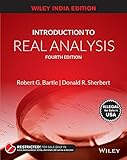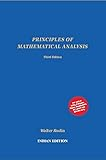# CSIR-NET: Analysis/Topology Quiz 2

Consider the interval (−1,1) and a sequence of elements in it. Then
Every limit point of is in (−1,1)
Consider the sequence . Is this sequence contained in (-1,1)? Where does it converge to?
Every limit point of is in [−1,1]
Note that closure of an open interval is the corresponding closed interval. And by definition, closure contains all the limit points of the set, hence this option.
The limit points of can only be in {−1,0,1}
Consider the sequence . Is this sequence contained in (-1,1)? Where does it converge to?
The limit points of cannot be in {−1,0,1}
Consider the sequence . Is this sequence contained in (-1,1)? Where does it converge to?

CSIR-NET June 2016, Part B Question.

How should I analyze this question when I am preparing for CSIR-NET?

1. Mark all mathematical terms here. Read their definitions. Note that if you had done this study for Quiz 1, you have already covered many definitions needed for this question and can save a lot of time by quickly recollecting them from your diary.
2. Try construct examples for each terms, try apply it in options. If there are any new examples you noticed, updated your diary.
3. This question is interesting because it talks about a lot of mathematical terms, but the keyword is nowhere mentioned in question – closure of a set. Read and analyse that definition, too.
4. Make a note of all your observations, examples that you found, in your NET preparation diary. For example, you should have asked questions like:
1.$\{0.5-\frac1n\}$ was constructed by manipulating the sequence$\{\frac1n\}$. Which all are some interesting sequences that I must keep in mind?
2. For which set will option 3 ({-1, 0, 1}) be closure of?
3. Should closure be unique?

Can you think of more questions? leave them in comment.

Watch the following video, discussing the same question:

Here are some suggested books for real analysis and topology, for NET/JRF Aspirants:

## Recommended Books for Real AnalysisIntroduction to Real Analysis by Bartle and Sherbert (4e)Principles of Mathematical Analysis by Rudin